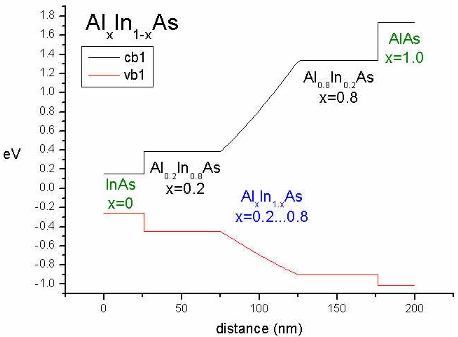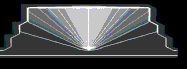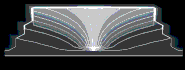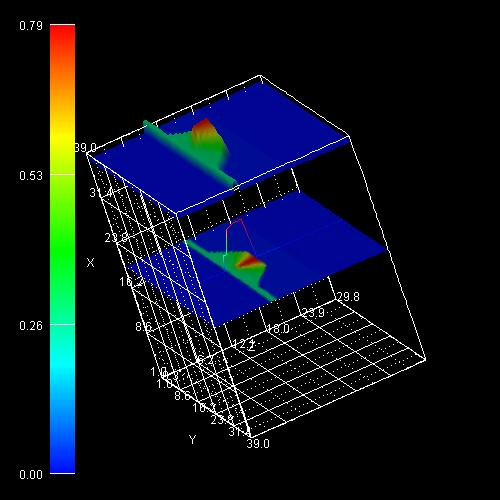nextnano.com  GUI: nextnanomat  Tool: nextnano++  Tool: nextnano³  Tool: nextnano.QCL  Download | Search | Copyright | News | Publications  * password protected nextnano³ softwareAlloy function

# alloy-function

## Specification of ternary alloy profile

For ternary materials, a name of an alloy function must be provided in the material specification.
The parameters for various built-in functions which generate the alloy profile have to be specified within the present keyword``` \$alloy-function```.

Note: Can be used with an alloy concentration sweep where the` xalloy `concentration is varied stepwise.

```!-----------------------------------------------------! \$alloy-function                              optional !  material-number              integer        required !  function-name                character      required !                                                       !  xalloy                       double         optional !  xalloy-minimum               double         optional ! ```for` gaussian`,` one-minus-gaussian`,``` biparabolic``````  xalloy-maximum               double         optional ! ```for` gaussian`,` one-minus-gaussian````  gauss-center                 double_array   optional ! ```for` gaussian`,` one-minus-gaussian````  gauss-width                  double_array   optional ! ```for` gaussian`,` one-minus-gaussian````  rotation-angles              double_array   optional ! ```for` gaussian`,` one-minus-gaussian`
```                                                      ! ```(probably some more later on)```  gauss-plane                  integer_array  optional ! ```for``` gaussia````n-2d`, e.g. (0,1,1)```  gauss-dir                    integer_array  optional ! ```for``` gaussia````n-1d`, e.g. (0,1,0)```  orientation                  integer_array  optional ! ```for` linear`, e.g. (0,1,0);```                                                       !```        `bilinear`, e.g. (1,1,0)
``` xalloy-from-to               double_array   optional ! ```for``` linear  vary-from-pos-to-pos         double_array   optional ! ```for``` linear  xalloy-at-corners            double_array   optional ! ```for` bilinear` (four values)```  position-corners             double_array   optional ! ```for` bilinear` (eight values, i.e. four```                                                       ! ```coordinate pairs in plane (orientation))```  Fermi-function-lambda        double         optional ! ```for Fermi function like alloy profile```  origin                       double_array   optional ! ```for inverse triangular, trumpet and onion profile in quantum dots (e.g. InGaAs), and for Fermi function```  trumpet-parameters           double_array   optional ! ```for trumpet shape alloy profile C1,C2,C3,alpha in quantum dots
``` onion-parameters             double_array   optional ! ```for onion    shape alloy profile C1,C2,C3,alpha in quantum dots
```                                                      !  filename-alloy-profile       character      optional ! ```for importing an alloy function```                                                       !  function                     character      optional ! ```for user-defined alloy function (new!)```                                                       !  alloy-sweep-active           character      optional ! ```for alloy sweep (new!)```  alloy-sweep-step-size        double         optional ! ```for alloy sweep (new!)```  alloy-sweep-number-of-steps  integer        optional ! ```for alloy sweep (new!)``` \$end_alloy-function                          optional ! !-----------------------------------------------------!```

`material-number = integer`
The material number of a ternary alloy material as given in the input file to which this function applies to.

```function-name = constant                           ! 1D,2D,3D               = linear                             ! 1D,2D,3D               = parabolic                          ! 1D,2D,3D```
```              = biparabolic                        !    2D,3D               = triparabolic                       !       3D               = Fermi-function                     ! 1D,2D,3D```
```              = gaussian-1d                        ! 1D,2D,3D               = gaussian-2d                        !    2D,3D               = gaussian-3d                        !       3D               = one-m-gaussian-1d                  ! 1D,2D,3D               = one-m-gaussian-2d                  !    2D,3D               = one-m-gaussian-3d                  !       3D               = bilinear                           !    2D,3D               = inv-triangle                       !       3D```
```              = trumpet                            !       3D```
```              = onion                              !       3D```
```              = import-alloy-profile               ! 1D,2D,3D               = alloy-profile-defined-by-function  ! 1D,2D,3D               = xy-gaussian-3d                     ! ```The '`xy-...`' functions are probably needed for quaternaries.```               = xy-gaussian-2d                     !               = xy-gaussian-1d                     !               = xy-one-m-gaussian-3d               !               = xy-one-m-gaussian-2d               !               = xy-one-m-gaussian-1d               !               = xy-constant                        !               = xy-linear                          !               = xy-bilinear                        ! ```
String is one of the predefined function names. Depending on the dimension of the simulation, various functions are available. The function names and their parameters are described in the following.

The x values of the alloys (Si1-xGex, AlxGa1-xAs, ...) are written out automatically and can be visualized:` alloy_grid1D.dat`

## One-dimensional simulations

```function-name = constant ```
```xalloy        = x          ! xalloy        = 0.2d0      ! e.g. Al(x)Ga(1-x)As, Al0.2Ga0.8As ```for constant alloy concentration
the constant `x` in AxB1-xC        ```0 <= x <= 1 ```Note: Can be used with an alloy concentration sweep where the` xalloy `concentration is varied stepwise.

```function-name        = linear orientation          = i j k ```(`0 0 1`) or (`0 1 0`) or (`1 0 0`) the alloy concentration varies along the selected simulation axis

```vary-from-pos-to-pos = pos1 pos2   !``` in units of``` [nm] ```Alloy concentration varies from` pos1 ` to``` pos2 ``` with respect to selected axis.``` pos1 < pos2 ```Example:``` vary-from-pos-to-pos = 75d0  125d0 ```
```xalloy-from-to       = x1 x2 x1``` = alloy concentration at ``` pos1```, `x2` = alloy concentration at `pos2`,
Outside the values (`pos1, pos2`) it is constant as specified by the boundary values (`x1, x2`).
Example:``` xalloy-from-to = 0.2d0  0.8d0  ```This conduction and valence band plot was generated by specifying: Material 1 (cluster-numbers = 1):           0 nm   -    25 nm Material 2 (cluster-numbers = 2):          25 nm   -   175 nm Material 3 (cluster-numbers = 3):        175 nm   -   200 nm but ` vary-from-pos-to-pos`:        75 nm   -   150 nm    (`pos1` - `pos2`) `---> `  Outside the values `pos1` and ``` pos2``` the boundary values `x1`=0.2 and ``` x2```=0.8 are taken (between  25-75  and  125-175).``` \$material  material-number = 1  material-name   = Al(x)In(1-x)As  cluster-numbers = 1  alloy-function  = constant  material-number = 2  material-name   = Al(x)In(1-x)As  cluster-numbers = 2  alloy-function  = linear  material-number = 3  material-name   = Al(x)In(1-x)As  cluster-numbers = 3  alloy-function  = constant \$end_material``` ``` \$alloy-function  material-number      = 1  function-name        = constant  xalloy               = 0.0d0             ! -> InAs  material-number      = 2  function-name        = linear  orientation          = 0 0 1  vary-from-pos-to-pos = 75d0 125d0  xalloy-from-to       = 0.2d0 0.8d0       ! -> from: Al0.2In0.8As                                           ! -> to:   Al0.8In0.2As  material-number      = 3  function-name        = constant  xalloy               = 1.0d0             ! -> AlAs \$end_alloy-function```

```function-name        = parabolic orientation          = i j k ```(`0 0 1`) or (`0 1 0`) or (`1 0 0`) the alloy concentration varies along the selected simulation axis

```vary-from-pos-to-pos = pos1 pos2   !``` in units of``` [nm] ```Alloy concentration varies from` pos1 ` to``` pos2 ``` with respect to selected axis.``` pos1 < pos2 ```Example:``` vary-from-pos-to-pos = 75d0  125d0 ```
```xalloy-from-to       = xmin xmax   ! xmin < xmax ``` and``` xmin > xmax ``` are allowed.``` xmin``` = alloy concentration at extremum of parabola, `xmax` = alloy concentration at positions``` pos1 ```and` pos2`.
Outside the values (`pos1, pos2`) it is constant as specified by the boundary values (`xmax`).
Example:``` xalloy-from-to = 0.2d0  0.8d0 ```Note: The origin of the parabola is set to: (```pos2 - ````pos1`) / 2.

For more details on the parabolic alloy profile, have a look in the Parabolic quantum well tutorial.

```function-name         = Fermi-function orientation           = i j k ```(`0 0 1`) or (`0 1 0`) or (`1 0 0`) the alloy concentration varies along the selected simulation axis

```vary-from-pos-to-pos  = pos1 pos2   !``` in units of``` [nm] ```Alloy concentration varies from` pos1 ` to``` pos2 ``` with respect to selected axis.``` pos1 < pos2 ```Example:``` vary-from-pos-to-pos = 75d0  125d0 ```
```xalloy-from-to        = xmin xmax   ! xmin < xmax ``` and``` xmin > xmax ``` are allowed.``` xmin``` = alloy concentration at position``` pos1```,
`xmax` = alloy concentration at position``` pos2```.
Outside the values (`pos1, pos2`) it is constant as specified by the boundary values (`xmin `or` xmax`, respectively).
Example:``` xalloy-from-to = 0.2d0  0.8d0 origin                = pos_center  ! ```center coordinate of the Fermi function in units of``` [nm] (pos1 < pos_center < pos2) ```Note: Even in 2D or 3D only one coordinate is expected (i.e. the one that corresponds to the digit` 1 `in``` orientation = i j k```).``` Fermi-function-lambda = 0.25d0 ! ```determines steepness of Fermi function, the smaller the number, the steeper the Fermi function``` ```An example of a Fermi function like alloy profile is shown in this figure.The quantum well is not assumed to be rectangular (as it is usually the case). It has a Fermi function like alloy profile (i.e. AlxGa1-xAs where x varies like a Fermi function) at the material interfaces (one Fermi function at the left, and one at the right material interface).
The origin of the left Fermi function is at 17.5 nm, the origin of the right Fermi function is at 32.5 nm.
The steepness parameter has been set to 0.25 (```Fermi-function-lambda = 0.25d0```).
If you want to obtain the input file for the Fermi function like quantum well (`1DFermiFunctionLikeAlloyProfile.in`), please contact stefan.birner@nextnano.de.

```function-name  = gaussian-1d gauss-dir      = i j k ```(`0 0 1`) or (`0 1 0`) or (`1 0 0`) the alloy concentration varies along the selected simulation axis

```gauss-center   = pos1 pos1``` = position of Gauss center (position of` xmax`) along direction specified by` gauss-dir`

```gauss-width    = sigma gauss-width ```is usually called sigma in the formula of the Gaussian distribution function.
For the meaning of``` gauss-width ```have a look at the 10 DM banknote of the German "Deutsche Mark" or any mathematical textbook.

```xalloy-minimum = xmin ```minimum value of alloy concentration

```xalloy-maximum = xmax ```maximum value of alloy concentration

```function-name  = one-m-gaussian-1d ```
```gauss-dir      = i j k ```(`0 0 1`) or (`0 1 0`) or (`1 0 0`) the alloy concentration varies along the selected simulation axis

`gauss-center   = pos1`
`pos1` = position of gauss center (position of ``` xmin```) along direction specified by` gauss-dir`

```gauss-width    = sigma ```For the meaning of``` sigma ```have a look at the 10 DM banknote of the German "Deutsche Mark" or any mathematical textbook.

```xalloy-minimum = xmin ```minimum value of alloy concentration

```xalloy-maximum = xmax ```maximum value of alloy concentration

## Two-dimensional simulations

All of the one dimensional functions can be used to generate profiles which are constant along one coordinate axis. Additionally we have

```function-name   = gaussian-2d gauss-plane     = i j k       ! ```specifies the plane in which the alloy concentration varies, e.g.``` 0 1 1 gauss-center    = pos1 pos2   ! ```specifies the coordinates of the gauss maximum with
```                              ! ```respect to the coordinate plane selected``` gauss-width     = sig1 sig2   ! ```widths of gaussian along the directions selected by
```                              ! ```gauss-plane``` xalloy-minimum  = xmin        ! ``` minimum value of alloy concentration``` xalloy-maximum  = xmax        ! ``` maximum value of alloy concentration``` rotation-angles = angle       ! ```rotation angle around the axis perpendicular to
```                              ! ```gauss-plane in``` [rad]  ```

```function-name   = one-m-gaussian-2d gauss-plane     = i j k              ! ```specifies the plane in which the alloy concentration varies, e.g.``` 0 1 1 gauss-center    = pos1 pos2          ! ```specifies the coordinates of the gauss minimum
```                                     ! ```with respect to the coordinate plane selected``` gauss-width     = sig1 sig2          ! ```widths of gaussian along the directions
```                                     ! ```selected by gauss-plane``` xalloy-minimum  = xmin               ! ```minimum value of alloy concentration``` xalloy-maximum  = xmax               ! ```maximum value of alloy concentration``` rotation-angles = angle              ! ```rotation angle around the axis perpendicular to
```                                     ! ```gauss-plane in``` [rad]  ```

```function-name     = bilinear      ! orientation       = i j k         ! ```variation of alloy concentration in this coordinate plane, e.g.``` 1 0 1 position-corners  = pos1 ... pos8 ! ```four pairs of coordinates for corners of a rectangle in plane selected by orientation``` ```Four coordinate pairs (8 numbers) are expected with the following restrictions:
```x1,y1 (1)   x2,y1 (2)   x2,y2 (3)    x1,y2 (4) ```Same names indicate equal numbers!``` x,y ```pairs refer to the plane in which the alloy concentration varies.
``` ----------------------------------- |```
```x1,y2 (4)                 x2,y2 (3)| |                                   | |                                   | |                                   | |x1,y1 (1)                 x2,y1 (2)|  -----------------------------------```

`xalloy-at-corners = x1 ... x4     ! `the alloy concentration at the corners defined above

```function-name     = biparabolic   ! orientation       = i j k         ! ```variation of alloy concentration in this coordinate plane, e.g.``` 1 0 1 position-corners  = pos1 ... pos8 ! ```four pairs of coordinates for corners of a rectangle in plane selected by orientation``` ```Four coordinate pairs (8 numbers) are expected with the following restrictions:
```x1,y1 (1)   x2,y1 (2)   x2,y2 (3)    x1,y2 (4) ```Same names indicate equal numbers!``` x,y ```pairs refer to the plane in which the alloy concentration varies.
``` ----------------------------------- |```
```x1,y2 (4)                 x2,y2 (3)| |                                   | |                                   | |                                   | |x1,y1 (1)                 x2,y1 (2)|  -----------------------------------```

`xalloy-at-corners = x1 ... x4     ! `the alloy concentration at the corners defined above

```xalloy-minimum    = xmin          ! ```minimum value of alloy concentration in the center of the rectangle

For more details on the biparabolic alloy profile, have a look in the tutorial: Fock-Darwin states of a 2D parabolic potential in a magnetic field.

## Three-dimensional simulations

All of the one- and two-dimensional functions can be used to generate alloy profiles. Additionally we have:

```function-name     = triparabolic   ! ```(can be used for quantum dots for example)``` position-corners  = pos1 ... pos16 ! ``` eight times three coordinates (xi, yi, zi) for corners of a cuboid (i.e. 24 numbers)``` ```To understand the restrictions, have a look at this example:
```position-corners  = 10d0 10d0 10d0 ! (x1,y1,z1), corner (1)                     20d0 10d0 10d0 ! (x2,y1,z1), corner (2)                     20d0 20d0 10d0 ! (x2,y2,z1), corner (3)                     10d0 20d0 10d0 ! (x1,y2,z1), corner (4)                     10d0 10d0 20d0 ! (x1,y1,z2), corner (5)                     20d0 10d0 20d0 ! (x2,y1,z2), corner (6)                     20d0 20d0 20d0 ! (x2,y2,z2), corner (7)                     10d0 20d0 20d0 ! (x1,y2,z2), corner (8) xalloy-at-corners = x1 ... x8     ! ```the alloy concentration at the corners defined above (at present, all values must be equal)

```xalloy-minimum    = xmin          ! ```minimum value of alloy concentration in the center of the cuboid

For more details on the triparabolic alloy profile, have a look at the biparabolic alloy profile in the tutorial: Fock-Darwin states of a 2D parabolic potential in a magnetic field or contact stefan.birner@nextnano.de for an example input file.

```function-name   = gaussian-3d    ! gauss-center    = pos1 pos2 pos3 ! ```specifies the coordinates of the gauss maximum
```                                 ! ```with respect to the coordinate plane selected``` gauss-width     = sig1 sig2 sig3 ! ```widths of gaussian along the directions selected by
```                                 ! ```gauss-plane``` xalloy-minimum  = xmin           ! ```minimum value of alloy concentration``` xalloy-maximum  = xmax           ! ```maximum value of alloy concentration``` rotation-angles = angle1 angle2  ! ```rotation angle around the axis perpendicular to
```                                 ! ```gauss-plane in``` [rad]  ```

```function-name   = one-m-gaussian-3d ! gauss-center    = pos1 pos2 pos3    ! ``` specifies the coordinates of the gauss minimum
```                                    ! ```with respect to the coordinate plane selected``` gauss-width     = sig1 sig2 sig3    ! ``` widths of gaussian along the directions selected
```                                    ! ```by gauss-plane``` xalloy-minimum  = xmin              ! ```minimum value of alloy concentration``` xalloy-maximum  = xmax              ! ```maximum value of alloy concentration``` rotation-angles = ang1 ang2 ang3    ! ```Euler rotation angles in` [rad]`

### Quantum dots

For a quantum dot, one can take e.g. four different alloy profiles:

 constant linear alloy profile inverted pyramid alloy profile trumpet alloy profile (trivial)```\$material  ...  alloy-function = constant  ... \$alloy-function  ...  function-name  = constant  xalloy         = 0.30d0  ``` ```\$material  ...  alloy-function = linear  ... \$alloy-function  ...  function-name  = linear  orientation    = 0 0 1  vary-from-pos-to-pos = z1d0 z2d0  xalloy-from-to = 0.30d0 0.70d0``` ```\$material  ...  alloy-function = inv-triangle  ... \$alloy-function  ...  function-name  = inv-triangle  origin         = xd0 yd0 zd0  xalloy-from-to = 0.2d0 0.8d0  ``` ```\$material  ...  alloy-function = trumpet  ... \$alloy-function  ...  function-name  = trumpet  origin         = x y z  trumpet-parameters = ...  ```

```origin             = 20d0  20d0  11d0  ! ```origin of apex located at point``` (x,y,z)=(20 nm, 20 nm, 11 nm) ```for inverse triangular and trumpet profile in quantum dots (e.g. InGaAs)

`alloy-function = inv-triangle`

`      `This formula considers an additional lateral variation of the indium content.
```      x = xmax - ( xmax - xmin ) cos2(phi)       ```where` phi `is the angle to the center axis. The formula is based on the model proposed by Tersoff (N. Liu et al., PRL 84, 334 (2000)).```       ```For simplicity the alloy profile is still isotropic around the center axis of the quantum dot.```       ```The indium content depends solely on the angle to the center axis,
`      `with high indium content for small angles as indicated by the light regions in the figure shown below.```  ```

`alloy-function = ``trumpet`

```trumpet-parameters = C1   C2   0.3d0   0.8d0  !  C1   C2   C3  alpha```for trumpet shape alloy profile``` C1```,``` C2```,` C3`,``` alpha ```in quantum dots. The minimum and maximum indium concentrations are given by
```xmax = 1 - C1```
```xmin = 1 - C2```

The parameters` C3 `and``` alpha ```can be used to vary the shape of the alloy profile while keeping the average indium content fixed. (M. Sabathil used``` C3 = 0.3 ```and``` alpha = 0.8 ```for InGaAs dots) .

The symmetry center is set by the specifier``` origin```.

Note: So far it only works for the growth direction being the z axis.

The indium concentration is given by this formula:

`    rho = SQRT(x2 + y2)`

```    f(x,y,z) = 1 - [ C2 + (C1 - C2) exp [ ( - rho  exp (- 2 z C3) ) / {alpha} ] ] =              =  xmin + ( xmax - xmin) exp [ ( - rho  exp (- 2 z C3) ) / {alpha} ]  ```

``` ```The formula is based on the more refined model proposed by Migliorato (M.A. Migliorato et al., PRB 65, 115316 (2002)).``` ```This profile resembles the horn of a trumpet and is thus called``` trumpet```.``` ```The maximum indium concentration is in the center axis of the quantum dot.

Example

• Quantum dot with inverse triangular alloy shape ("inverted pyramid")
```\$material                            !  ...  material-number = 5                 !  material-name   = In(x)Ga(1-x)As    ! ```InGaAs quantum dot```  cluster-numbers = 5                 !  alloy-function  = inv-triangle      !  ... \$end_material                        ! \$alloy-function                      !  ...  material-number = 5                 !  function-name   = inv-triangle      !  origin          = 20d0  20d0  11d0  ! ```origin of apex located at point` (x,y,z)=(20,20,11) `(top of inverted pyramid)```  xalloy-from-to  = 0.28d0    0.80d0  ! ```vary alloy concentration from apex/origin (`0.28`: In0.28Ga0.72As)```                                      ! ```to bottom of inverted pyramid (`0.80`: In0.80Ga0.20As)```  ... \$end_alloy-function ```This is a plot of the conduction band of an inverted pyramid-like alloy profile inside a quantum dot:
Red (80 % In): In0.80Ga0.20As``` ```Green (28 % In): In0.28Ga0.72As``` ```### Onion profile for quantum dots

Thanks to T. Fromherz, University of Linz, for implementing the function``` onion```.

`alloy-function = onion`

```onion-parameters = 0.2d0  C2   C3   0d0    !  C1   C2   C3  alpha```for onion shape alloy profile``` C1```,``` C2```,` C3`,``` alpha ```in quantum dots.

` C1`: background alloy concentration             in units of` []`
` C2`:       vertical alloy concentration variation in units of` [nm-0.5]`
` C3`:        lateral alloy concentration variation in units of` [nm-2]`
(`alpha`: currently not used but this dummy parameter has to be present)

The alloy concentration is given by this formula where` x,y,z ` refer to the simulation coordinate system in units of` [nm]`:

`rho = SQRT(x2 + y2)`

```alloyc = C1 + C2 * SQRT(z) + C3 * rho2```

The symmetry center is set by the specifier``` origin```.

Note: So far it only works for the growth direction being the z axis.

Example

• Quantum dot with onion alloy shape
(...has to be added)

### User-defined alloy function (new)

Using the alloy function` alloy``-profile-defined-by-function`, one can define an arbitrary function n(x,y,z) for the alloy profile.
The variables x, y, z refer to the grid point coordinates of the simulation area in units of` [nm]`.

Example: A parabolic alloy profile.

```\$material  ...  alloy-function = alloy-profile-defined-by-function  ... \$alloy-function  ...  %K = 0.02  function-name  = alloy-profile-defined-by-function  function       = " 1/2 * %K * (z-15)^2 "            ! alloy(x,y,z) = ... ```In this example, the parabolic alloy function profile is shifted by 15 nm along the z axis.

The following operators and functions are supported:
```+ , - , * , / , ^ abs , exp , sqrt , log , log10 , sin , cos , tan , sinh , cosh , tanh , asin , acos , atan```

If the function leads to a value smaller than 0.0, then 0.0 is taken for the alloy concentration.
If the function leads to a value larger than 1.0, then 1.0 is taken for the alloy concentration.

## Reading in alloy profiles from a file

```alloy-function = import-alloy-profile ```
```filename-alloy-profile = "D:\nextnano simulations\alloy_function.dat"```
```                       =  D:\nextnano_simulations\alloy_function.dat```
alloy profile filename to be read in (e.g. experimental values). The string can include a folder name.
The ASCII file must contain 2 (1D), 3 (2D) or 4 (3D) columns in each line:

1D:``` x coordinate [nm]                                            alloy concentration ...                                                          ...```

2D:``` x coordinate [nm]    y coordinate [nm]                       alloy concentration ...                  ...                                     ...```

3D:``` x coordinate [nm]    y coordinate [nm]   z coordinate [nm]   alloy concentration ...                  ...                 ...                 ...```

It must hold:``` 0 <= alloy concentration <= 1 ```The first line of this ASCII file can contain an optional header line with column descriptors.

If you want to obtain input files that show how to import an alloy profile from a file
`1DFermiFunctionLikeAlloyProfile_read_in_alloy_profile.in`
`2D_InGaAs_GaAs_QW_arbitrary_alloy_profile_xz_rect.in`

## Alloy concentration sweep

It is possible to sweep over the alloy concentration, i.e. to vary the alloy concentration stepwise.
In each alloy sweep step, the specifier` xalloy `(```constant ```alloy function)` `is increased by` alloy-sweep-step-size`.
The output is labelled by` ..._ind000.dat`,` ..._ind001.dat`,``` ... ```for each alloy sweep step.

``` alloy-sweep-active           = yes       ! ```to switch on alloy sweep```                               = no        ! ```to switch off alloy sweep
``` alloy-sweep-step-size        = 0.05d0    ! ```increase alloy concentration in each step by 0.05```                                           ! ```(This value can also be negative.)```  alloy-sweep-number-of-steps  = 5         ! ```number of alloy sweep steps

Restrictions:
`- `Voltage sweeps (`\$voltage-sweep`) and other sweeps cannot be combined with alloy sweeps at present.
`- `Only one alloy sweep is allowed at present.

## For code developers

If you want to add new alloy functions to the code, you will have to modify these files:

• ``` database_nn3.in```

```==> \$known-function-names```
`==> `Simply add name of the new alloy function in an analogous manner. This is trivial.
• ```input_parser / alloy / allocate_alloy_structures.f90 ==> ```Simply add name of the new alloy function in an analogous manner. This is trivial.
• ```input_parser / alloy / alloy_param_type.f90 ==> ```Simply add new MODULE and variables of the new alloy function in an analogous manner. This is easy.
• ```input_parser / read_profile_parameters / read_alloy_data.f90 ==> ```Simply add name of the new alloy function in an analogous manner. This is trivial.
• ```input_parser / read_profile_parameters / read_para_constant.f90 ```(Here:``` constant ```alloy function)``` ==> ```Simply add new MODULE that reads in the specifiers of the new alloy function from the input file and assigns the values to the relevant variables (in an analogous manner). This is not difficult.
• ```input_parser / treat_profiles / alloy.f90 ==> ```Simply add name of the new alloy function in an analogous manner. This is trivial.
• ```input_parser / treat_profiles / constant.f90 ==> ```Simply add the equations that calculate the alloy concentration (as a function of grid points) of the new alloy function in an analogous manner.

That's it!
Don't forget to test your new alloy function thoroughly in 1D, 2D and 3D,
as well as for different orientations:
` - `1D: growth along x, y or z
` - `2D: (x,y) plane, (y,z) plane, (x,z) plane)
` - `3D: is okay (only one orientation is possible)